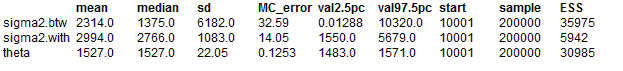#### Dyes: variance components model

Box and Tiao (1973) analyse data first presented by Davies (1967) concerning batch to batch variation in yields of dyestuff. The data (shown below) arise from a balanced experiment whereby the total product yield was determined for 5 samples from each of 6 randomly chosen batches of raw material.The object of the study was to determine the relative importance of between batch variation versus variation due to sampling and analytic errors. On the assumption that the batches and samples vary independently, and contribute additively to the total error variance, we may assume the following model for dyestuff yield:

yij ~ Normal(μi, τwithin)

μi ~ Normal(θ, τbetween)

where yij is the yield for sample j of batch i, μi is the true yield for batch i, τwithin is the inverse of the within-batch variance σ2within ( i.e. the variation due to sampling and analytic error), θ is the true average yield for all batches and τbetween is the inverse of the between-batch variance s2between. The total variation in product yield is thus σ2total = σ2within + σ2between and the relative contributions of each component to the total variance are fwithin = σ2within / σ2total and fbetween = σ2between / σ2total . We assume standard non-informative priors for θ, τwithin and τbetween.

Graphical model for dyes exampleBugs language for dyes example

model
{
for(i in 1 : batches) {
mu[i] ~ dnorm(theta, tau.btw)
for(j in 1 : samples) {
y[i , j] ~ dnorm(mu[i], tau.with)
}
}
sigma2.with <- 1 / tau.with
sigma2.btw <- 1 / tau.btw
tau.with ~ dgamma(0.001, 0.001)
tau.btw ~ dgamma(0.001, 0.001)
theta ~ dnorm(0.0, 1.0E-10)
}
##### Data
``` list(batches = 6, samples = 5,      y = structure(      .Data = c(1545, 1440, 1440, 1520, 1580,1540, 1555, 1490, 1560, 1495,1595, 1550, 1605, 1510, 1560,1445, 1440, 1595, 1465, 1545,1595, 1630, 1515, 1635, 1625,1520, 1455, 1450, 1480, 1445), .Dim = c(6, 5))) ```
##### Inits for chain 1
``` list(theta=1500, tau.with=1, tau.btw=1) ```

##### Inits for chain 2
``` list(theta=3000, tau.with=0.1, tau.btw=0.1) ```
ResultsNote that a relatively long run was required because of the high autocorrelation between successively sampled values of some parameters. Such correlations reduce the 'effective' size of the posterior sample, and hence a longer run is needed to ensure sufficient precision of the posterior estimates. Note that the posterior distribution for σ2between has a very long upper tail: hence the posterior mean is considerably larger than the median. Box and Tiao estimate σ2within = 2451 and σ2between = 1764 by classical analysis of variance. Here, σ2between is estimated by the difference of the between- and within-batch mean squares divided by the number of batches - 1. In cases where the between-batch mean square within-batch mean square, this leads to the unsatisfactory situation of a negative variance estimate. Computing a confidence interval for σ2between is also difficult using the classical approach due to its complicated sampling distribution